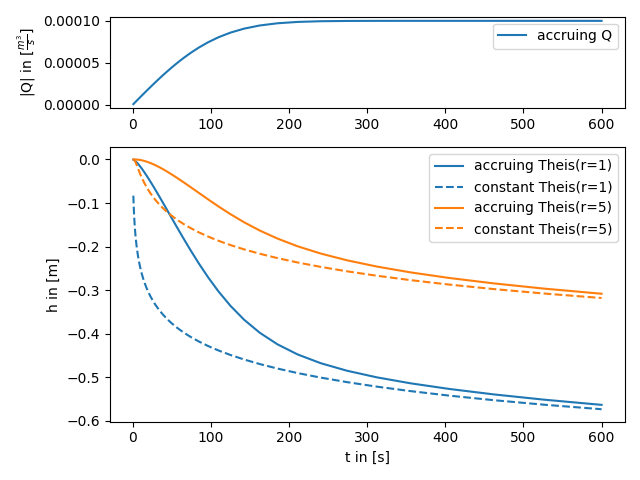# Accruing pumping rate

AnaFlow provides different representations for the pumping condition. One is an accruing pumping rate represented by the error function. This could be interpreted as that the water pump needs a certain time to reach its constant rate state.import matplotlib.gridspec as gridspec
import numpy as np
from matplotlib import pyplot as plt
from scipy.special import erf

from anaflow import theis

time = np.geomspace(1, 600)

# Q(t) = Q * erf(t / a)
a = 120
lap_kwargs = {"cond": 4, "cond_kw": {"a": a}}

time=time,
storage=1e-4,
transmissivity=1e-4,
rate=-1e-4,
lap_kwargs=lap_kwargs,
)
time=time,
storage=1e-4,
transmissivity=1e-4,
rate=-1e-4,
)
gs = gridspec.GridSpec(2, 1, height_ratios=[1, 3])
ax1 = plt.subplot(gs)
ax2 = plt.subplot(gs, sharex=ax1)

ax2.plot(
time,
color="C" + str(i),
label="accruing Theis(r={})".format(step),
)
ax2.plot(
time,
color="C" + str(i),
label="constant Theis(r={})".format(step),
linestyle="--",
)
ax1.plot(time, 1e-4 * erf(time / a), label="accruing Q")
ax2.set_xlabel("t in [s]")
ax2.set_ylabel("h in [m]")
ax1.set_ylabel(r"|Q| in [$\frac{m^3}{s}$]")
ax1.legend()
ax2.legend()
plt.tight_layout()
plt.show()


Total running time of the script: ( 0 minutes 0.257 seconds)

Gallery generated by Sphinx-Gallery